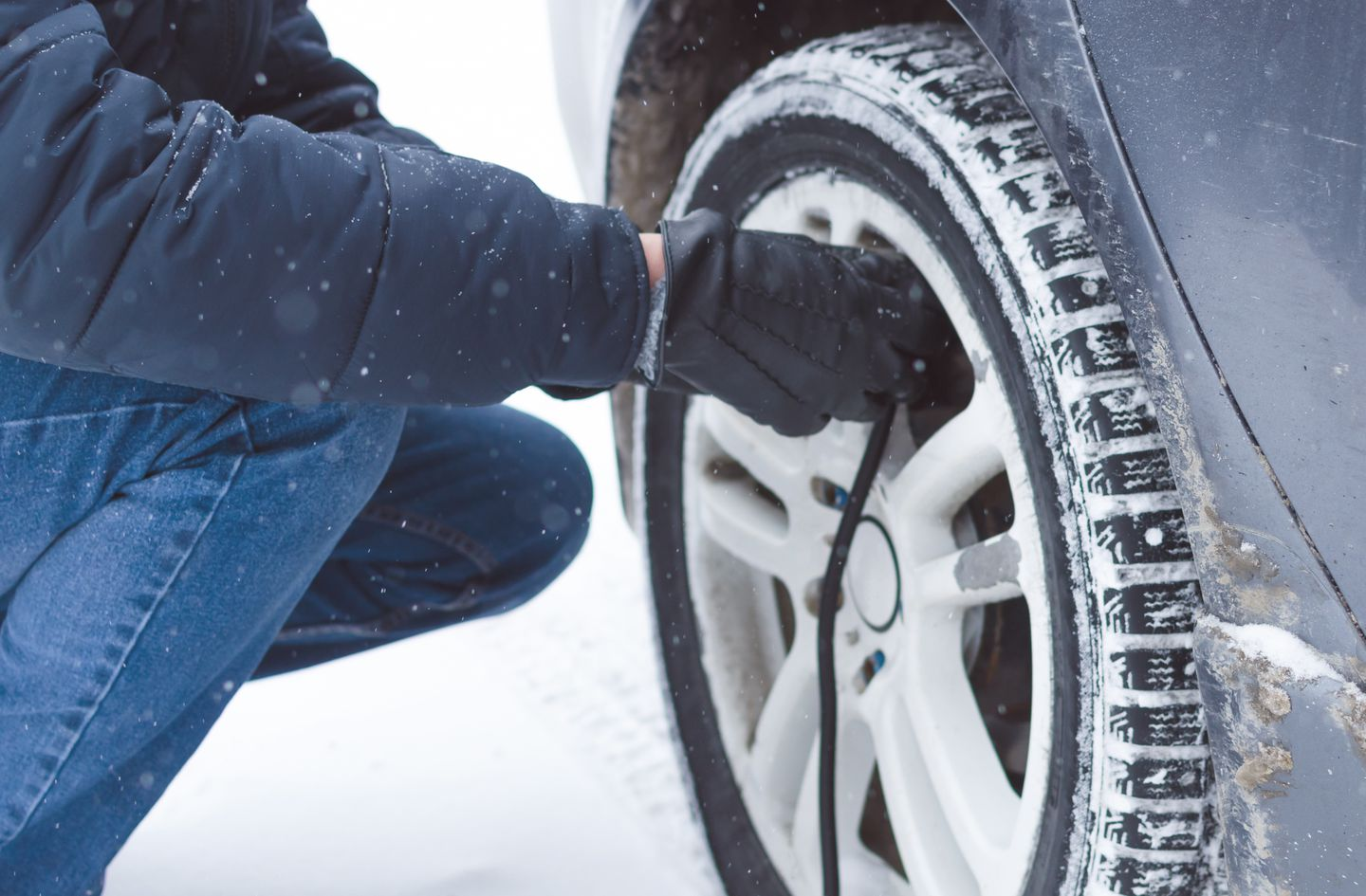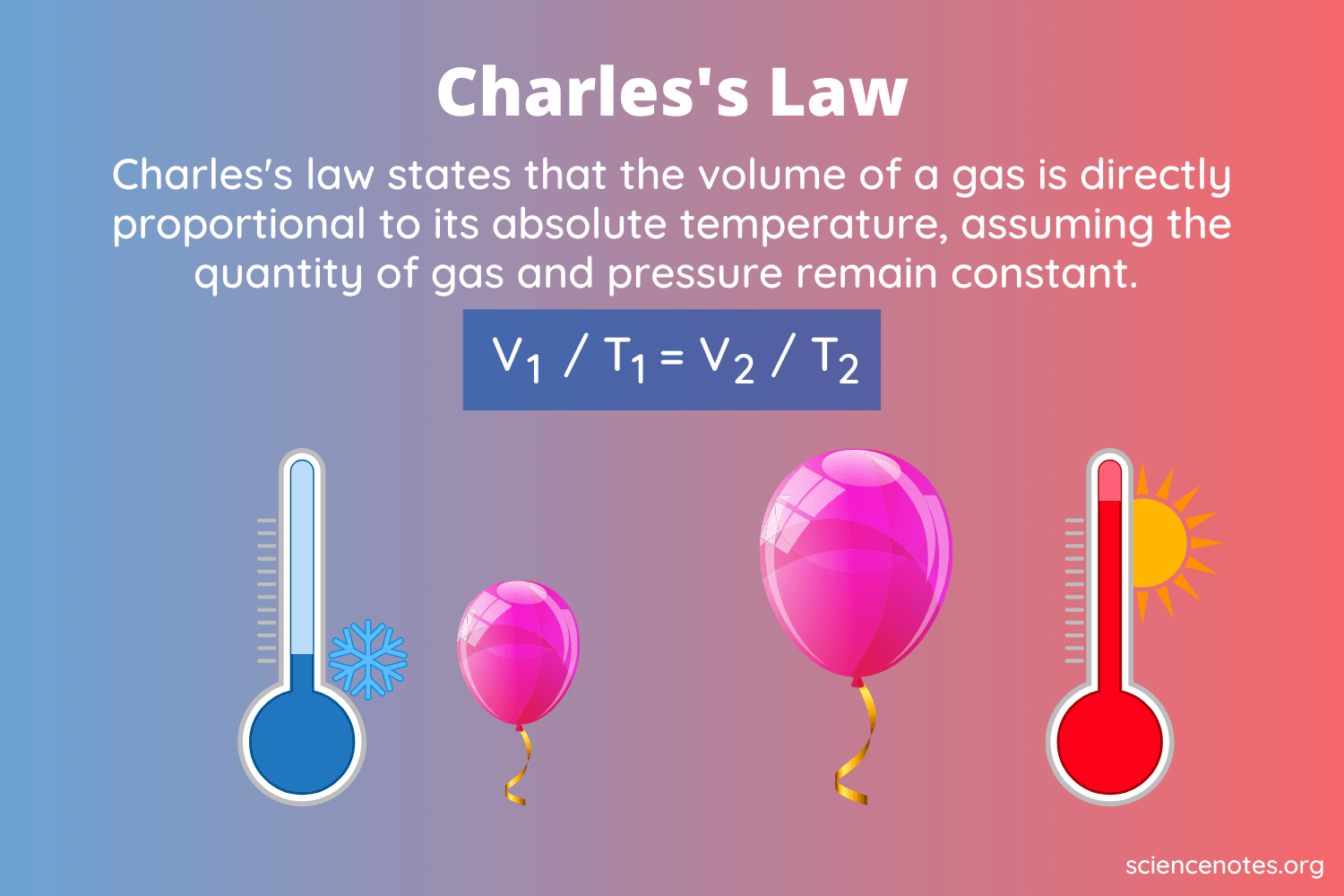Sarah Taylor

HS-PS1-6

# Charles's Law Study Guide

🗻 Big Picture: Charles's law, also known as the law of volume, gives us a detailed description of how gas expands at different temperatures.

### Lesson Objectives

• Define Charles’s Law
• Describe the relationship between temperature, T, and the volume, V, of its container with a constant amount of gas and pressure
• Solve problems using the Charles’s law equation
• Derive Charles’s law

## INTRODUCTIONSource

Everyone knows it’s important to check your tire pressure in the winter (and if you didn’t, you do now!). But what’s the science behind it? It all comes down to the relationship between temperature and pressure, which can be explained by Charles’s law. The law states that the volume of a gas is directly proportional to the absolute temperature at a constant pressure with a constant amount of gas particles.

In the winter, the temperature drops, causing the volume of gas contained within a tire to decrease. Underinflated tires have less precise cornering and handling, causing a bumpy, uncomfortable ride. Besides that, tires with low pressure have a lower fuel efficiency, so not only will you have a smoother ride with properly inflated tires, you’ll be saving cash too. Let’s talk more about the details of Charles’s law and how you can use it to calculate the temperature or volume of a gas when pressure and particle concentration are held constant.Source

### Charles’s Law Definition, Formula, & Derivation

Mathematically, Charles’s Law can be expressed as:

V ∝ T

Where:

• V = Volume
• T = Absolute temperature

Meaning that volume is proportional to temperature. Because Charles’s law states that pressure and the amount of gas remain constant, we can replace them with the constant k to translate it into the algebraic expression:

If we let the initial volume equal:

V1 ÷ T1 = k

and the final volume equal:

V2 ÷ T2 = k

We can combine these equations to form the Charles’s law equation:

V2 ÷ V1 = T2 ÷ T1 or V1T2 = V2T1

Where:

• V2 = final volume
• V1 = initial volume
• T2 = final absolute temperature
• T1 = initial absolute temperature

### Sample Problem: Charles’s Law

A balloon is filled to a volume of 4.3 L at a temperature of 25°C. The balloon is then heated to a temperature of 54°C. Given this information, what is the new volume of the balloon?

Step 1: Define the variables

• V1 = 4.3 L
• V2 = ?
• T1 = 25°C = 198.15 K
• T2 = 54°C = 327.15

For the purpose of this problem, let’s use V1T2 = V2T1

Step 3: Calculate

V1T2 = V2T1

(4.3 L)(327.15 K) = (V2 )( 198.15 K)

(1406.745 L/K)/198.15 K = V2

7.0994 = V2

The new volume of the balloon is 7.0994.

## 💡 Summary

• Charles’s law states that the volume of a gas is directly proportional to the absolute temperature at a constant pressure with a constant amount of gas particles.

• The Charles’s law equation is written as V2 ÷ V1 = T2 ÷ T1 or V1T2 = V2T1

### 🧐 FAQs

1. What does Charles's law state?

Charles's Law states that the volume of a gas is directly proportional to the absolute temperature at a constant pressure.

2. Write down Charles's Law formula.

Charles's Law formula is written as:

``````               VI /TI = VF/ TF
``````

Where:

VI = Initial volume VF = Final volume TI = Initial absolute temperature TF = Final absolute temperature

Here the temperatures are absolute temperatures that are measured in Kelvin, not in ⁰F or ⁰C.

3. What do Boyle's laws state?

Boyle's law states that a gas's pressure and volume are inversely proportional to each other.

We hope you enjoyed studying this lesson and learned something cool about Charles's Law and Boyle's Law! Join our Discord community to get any questions you may have answered and to engage with other students just like you! Don't forget to download our app to experience our fun VR classrooms - we promise it makes studying much more fun! 😎

## Sources

1. Charles’s Law of Ideal Gases: https://www.ck12.org/c/chemistry/charless-law/lecture/The-Sci-Guys:-Charless-Law-of-Ideal-Gases/. Accessed 3rd March 2022.
2. Boyle’s Law Examples: https://chemistrygod.com/boyle-law-examples. Accessed 3rd March 2022.